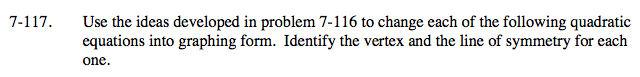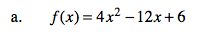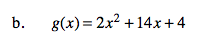### Home > A2C > Chapter 7 > Lesson 7.2.2 > Problem7-117

7-117.Make the coefficient of x² equal to 1 by factoring out a 4 in the first two terms.

Take half of '3' and square it. You get: (1.5)² = 2.25. The '4' indicates you really added 4(2.25) or 9. So add the 2.25 inside the ( )'s and subtract the equivalent '9' outside the ( )'s on the same side.

Then simplify.

f(x) = 4(x2 − 3x) + 6

f(x) = 4(x² − 3x + 2.25) + 6 −9

f(x) = 4(x − 1.5)2 − 3See part (a).